# C++ Program to Concatenate Two Strings

Here you will learn about and get code for string concatenation in C++. The program to concatenate strings is created in the following ways:

## String concatenation without strcat() in C++

This program concatenates or appends a second string to the first without using any library functions like strcat().

```#include<iostream>
#include<stdio.h>
using namespace std;
int main()
{
char strOne, strTwo, i, j=0;
cout<<"Enter the First String: ";
gets(strOne);
cout<<"Enter the Second String: ";
gets(strTwo);
for(i=0; strOne[i]!='\0'; i++)
j++;
for(i=0; strTwo[i]!='\0'; i++)
{
strOne[j] = strTwo[i];
j++;
}
strOne[j] = '\0';
cout<<"\nString after Concatenation: "<<strOne;
cout<<endl;
return 0;
}```

This program was built and runs under the Code::Blocks IDE. Here is its sample run: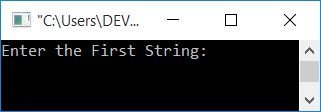Now supply any string as input, say codes, and then another string as input, say cracker. Press the `ENTER` key to add the second string "cracker" to the first "codes," as shown in the output given below: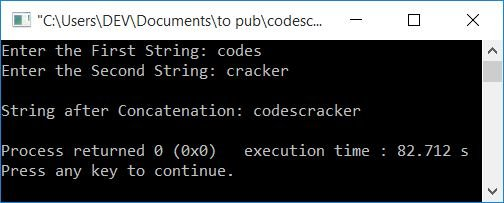Here is another sample run with user input; this is is the first string and codescracker is the second string: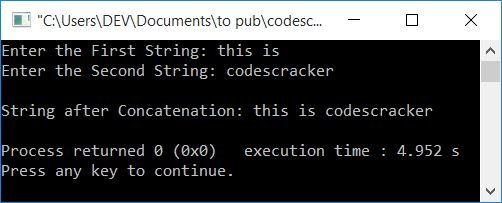Note: Don't forget to enter a space after this is or before codescracker while entering the first or second string. Otherwise both the strings gets concatenated without space like this iscodescracker.

The following two statements:

```strOne[j] = strTwo[i];
j++;```

can also be replaced with:

`strOne[j++] = strTwo[i];`

That is, a post increment to j is used, which increments its value by 1 after evaluating the statement:
`strOne[j] = strTwo[i];`

If the user enters the first string as codes, then it gets initialized to strOne in a way that:

• The first character (c) is set to strOne
• Similarly, strOne=o, strOne=d, strOne=e, strOne=s

And if the user enters cracker as a second string, then it also gets initialized to strTwo in a similar way. Therefore, with this two-string input, the dry run of the above program goes like this:

• At the first execution of the for loop, 0 gets initialized to i and checks the condition, strOne[i]!='\0', strOne!='\0', or c!='\0'.
• The condition is evaluated as true. Because c is not equal to a null-terminated character
• Therefore, program flow goes inside the loop and increments the value of j.
• Then the program flow goes back to the update part of the for loop, increments the value of i, and checks for the condition again with the new value of i.
• This process continues until the condition is evaluated as true.
• Because the first string is codes, at the fifth time, the condition evaluates to be false, and the program flow exits the loop and goes to second for loop. Now the value of j is 5.
• Therefore, from the 5th index, all the characters (one by one) of the second string gets initialized to the first string in a way that:
• The first character (c) of the second string gets initialized to strOne.
• The second character (r) of the second string gets initialized to strOne.
• Similarly, strOne = a, strOne = c, strOne = k, strOne = e, and strOne = r.
• After the loop is completed, \0 is initialized to strOne.
• Print the value of strOne that equals codescracker.

## String concatenation using strcat() in C++

The function strcat() takes two strings as arguments. The value of the second string gets appended or concatenated to the first string. For example, if there are two strings, say strOne and strTwo. And the value of both strings are:

```strOne = "codes";
strTwo = "cracker";```

Then,

`strcat(strOne, strTwo);`

That means strOne is now equal to codescracker. That is, the value of the second string concatenated to the first one. This function is defined in a string.h library.

```#include<iostream>
#include<stdio.h>
#include<string.h>
using namespace std;
int main()
{
char strOne, strTwo;
cout<<"Enter the First String: ";
gets(strOne);
cout<<"Enter the Second String: ";
gets(strTwo);
strcat(strOne, strTwo);
cout<<"\nString after Concatenation: "<<strOne;
cout<<endl;
return 0;
}```

This program will produce the same output as the previous one.

## String Concatenation using the + Operator in C++

The question is: write a program in C++ to concatenate two strings using the + operator. The answer to this question is given below:

```#include<iostream>
using namespace std;
int main()
{
string strOne="", strTwo="", strThree="";
cout<<"Enter the First String: ";
cin>>strOne;
cout<<"Enter the Second String: ";
cin>>strTwo;
strThree = strOne + strTwo;
cout<<"\nString after Concatenation: "<<strThree;
cout<<endl;
return 0;
}```

Here is its sample run with user input, codes, and cracker as first and second strings: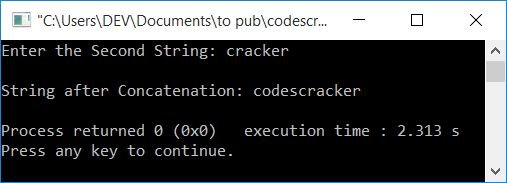Note: The previous program will only work with string input without any spaces.

## String Concatenation using a Pointer in C++

This is the last program that concatenates two strings using pointers.

```#include<iostream>
#include<stdio.h>
using namespace std;
int main()
{
char strOne, strTwo;
char *firstString, *secondString;
cout<<"Enter the First String: ";
gets(strOne);
cout<<"Enter the Second String: ";
gets(strTwo);
firstString = strOne;
secondString = strTwo;
while(*firstString)
firstString++;
while(*secondString)
{
*firstString = *secondString;
firstString++;
secondString++;
}
*firstString = '\0';
cout<<"\nString after Concatenation: "<<strOne;
cout<<endl;
return 0;
}```

Here's an example of how it works with user input, C++ Program to as the first string, and Concatenate Two Strings as the second string: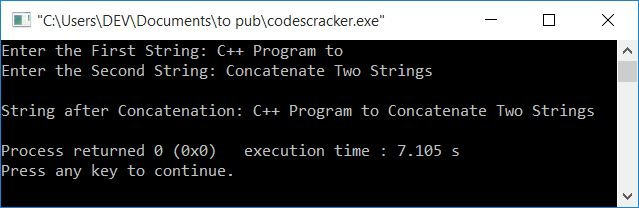The address of both the string strOne and strTwo gets initialized to its corresponding pointers, say firstString and secondString. And based on the address, we've concatenated the second string's value to the first one.

Note: The * is called as the value at operator.

#### The same program in different languages

C++ Quiz

« Previous Program Next Program »